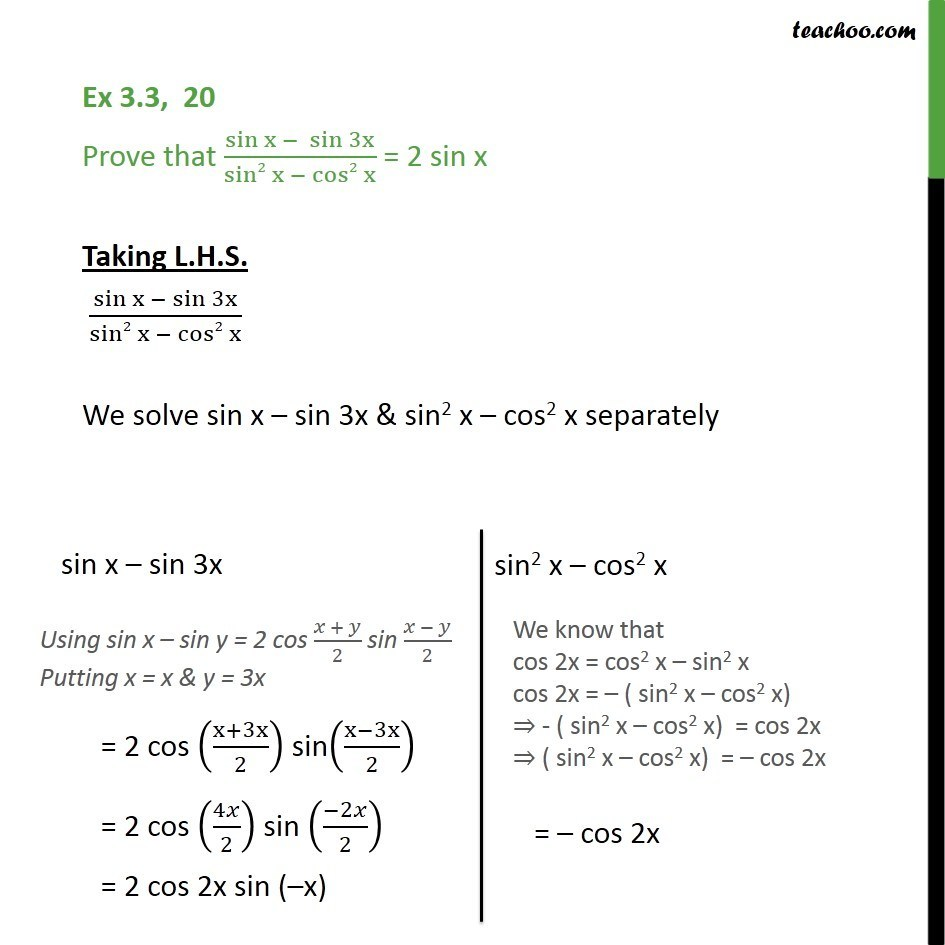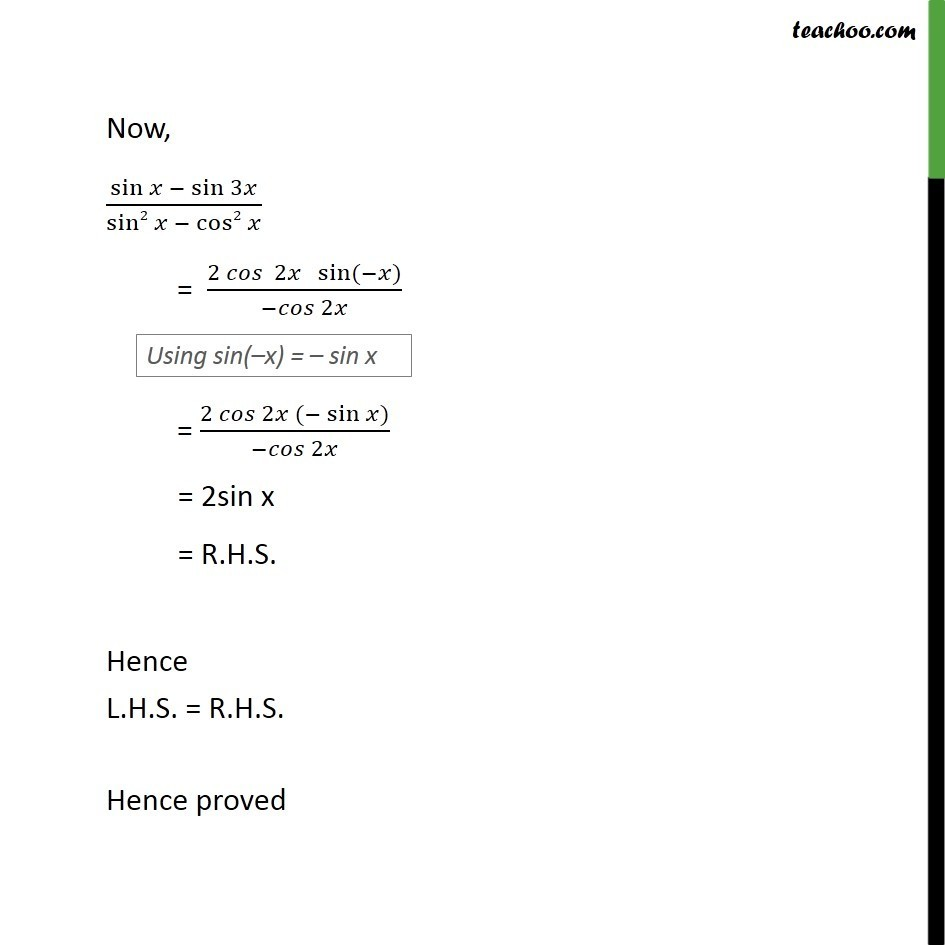Learn All Concepts of Chapter 2 Class 11 Relations and Function - FREE. Check - Trigonometry Class 11 - All Concepts1. Chapter 3 Class 11 Trigonometric Functions
2. Serial order wise
3. Ex 3.3

Transcript

Ex 3.3, 20 Prove that 〖sin x − 〗⁡sin⁡3x /(sin2⁡x − cos2⁡x ) = 2 sin x Taking L.H.S. 〖sin x −〗⁡sin⁡3x /(sin2⁡x − cos2⁡x ) We solve sin x – sin 3x & sin2 x – cos2 x separately Now, sin⁡〖𝑥 − sin⁡3𝑥 〗/sin2⁡〖𝑥 − cos2⁡𝑥 〗 = 〖2 𝑐𝑜𝑠 〗⁡〖2𝑥 〖 sin〗⁡〖(−𝑥)〗 〗/〖−𝑐𝑜𝑠〗⁡2𝑥 = 〖2 𝑐𝑜𝑠〗⁡〖2𝑥 〖(− sin〗⁡𝑥)〗/〖−𝑐𝑜𝑠〗⁡2𝑥 = 2sin x = R.H.S. Hence L.H.S. = R.H.S. Hence proved

Ex 3.3